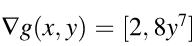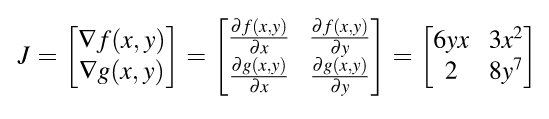BLOGIntroduction to vector calculus and partial derivatives

11-12-2018  0 Comment(s)

Neural network layers are not single functions of a single parameter, f(x). So, let’s move on to functions of multiple parameters such as f(x,y). For example, what is the derivative of xy (i.e., the multiplication of x and y)?

Well, it depends on whether we are changing x or y. We compute derivatives with respect to one variable (parameter) at a time, giving us two different partial derivatives for this two-parameter function (one for x and one for y). Instead of using operator d/dx, the partial derivative operator is ∂/ ∂x (a stylized d and not the Greek letter δ ). So ∂(xy)/ ∂x and ∂(xy)/ ∂y are the partial derivatives of xy; often, these are just called the partials.

The partial derivative with respect to x is just the usual scalar derivative, simply treating any other variable in the equation as a constant. Consider function f(x,y) = 3x²y. The partial derivative with respect to x is written ∂(3x²y)/ ∂x. There are three constants from the perspective of ∂/ ∂x: 3, 2, and y. Therefore, ∂(3x²y)/ ∂x = 3y∂(x²)/ ∂x = 3y(2x) = 6xy. The partial derivative with respect to y treats x like a constant and we get ∂(3x²y)/ ∂y = 3x².

Matrix calculus

When we move from derivatives of one function to derivatives of many functions, we move from the world of vector calculus to matrix calculus. Let us bring one more function g(x,y) = 2x + y⁸. So gradient of g(x,y) isGradient vectors organize all of the partial derivatives for a specific scalar function. If we have two functions, we can also organize their gradients into a matrix by stacking the gradients. When we do so, we get the Jacobian matrix(or just the Jacobian) where the gradients are rows:Comment Here